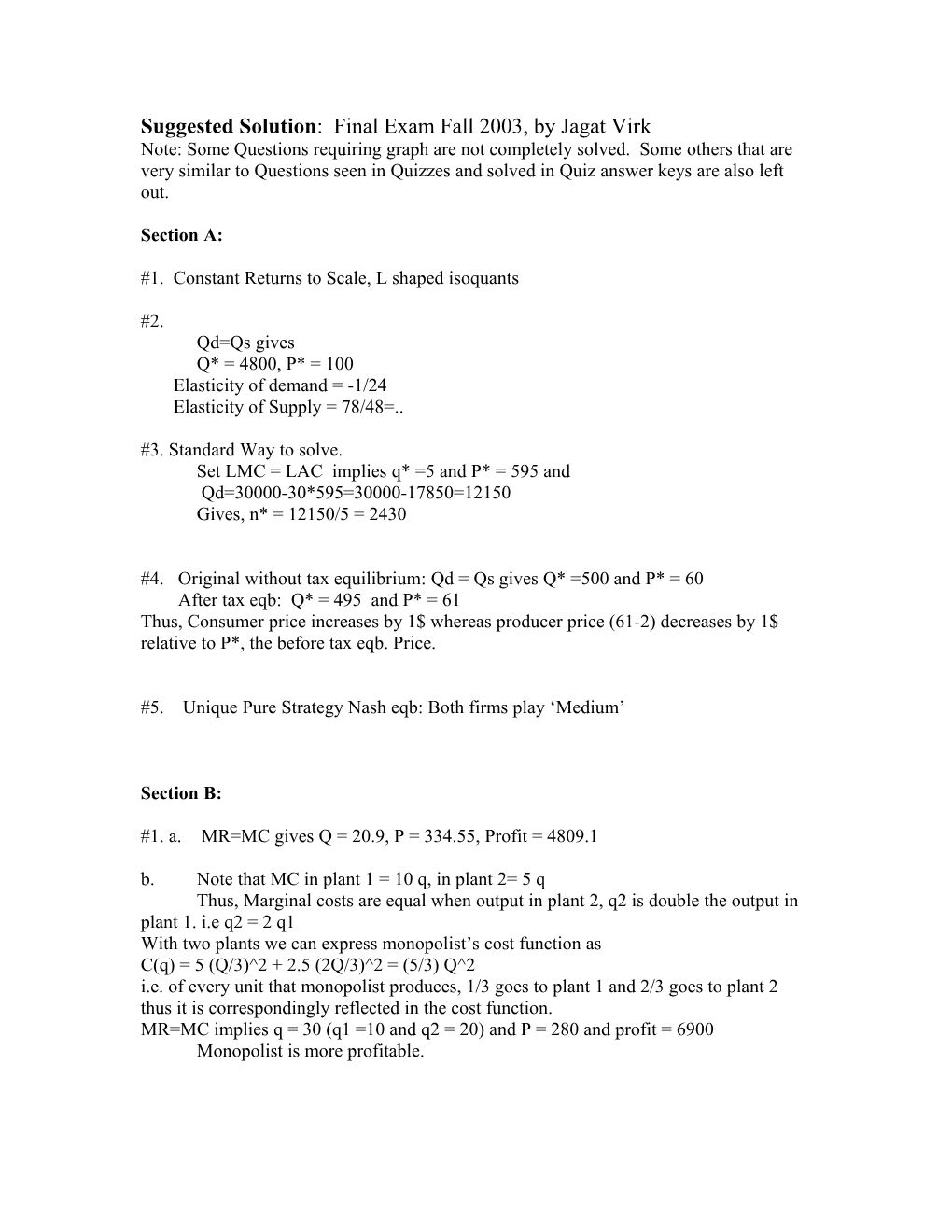# Suggested Solution: Final Exam Fall 2003, by Jagat VirkSuggested Solution: Final Exam Fall 2003, by Jagat Virk

Note: Some Questions requiring graph are not completely solved. Some others that are very similar to Questions seen in Quizzes and solved in Quiz answer keys are also left out.

Section A:

#1. Constant Returns to Scale, L shaped isoquants

#2.

Qd=Qs gives

Q* = 4800, P* = 100

Elasticity of demand = -1/24

Elasticity of Supply = 78/48=..

#3. Standard Way to solve.

Set LMC = LAC implies q* =5 and P* = 595 and

Qd=30000-30*595=30000-17850=12150

Gives, n* = 12150/5 = 2430

#4. Original without tax equilibrium: Qd = Qs gives Q* =500 and P* = 60

After tax eqb: Q* = 495 and P* = 61

Thus, Consumer price increases by 1\$ whereas producer price (61-2) decreases by 1\$ relative to P*, the before tax eqb. Price.

#5. Unique Pure Strategy Nash eqb: Both firms play ‘Medium’

Section B:

#1. a. MR=MC gives Q = 20.9, P = 334.55, Profit = 4809.1

b.Note that MC in plant 1 = 10 q, in plant 2= 5 q

Thus, Marginal costs are equal when output in plant 2, q2 is double the output in plant 1. i.e q2 = 2 q1

With two plants we can express monopolist’s cost function as

C(q) = 5 (Q/3)^2 + 2.5 (2Q/3)^2 = (5/3) Q^2

i.e. of every unit that monopolist produces, 1/3 goes to plant 1 and 2/3 goes to plant 2 thus it is correspondingly reflected in the cost function.

MR=MC implies q = 30 (q1 =10 and q2 = 20) and P = 280 and profit = 6900

Monopolist is more profitable.

1. No, Given the nature of Marginal cost functions it is optimal for monopolist to use first plant to produc one third of the profit maximizing production level.

#2. a. Tangency condition: (MPL/MPK) =K/L=w/r and production function gives

Q = 5 L^0.5 (wL/r)^0.5 = 5 L (w/r)^0.5

Implies L = Q/5 (r/w)^0.5 and K = Q/5 (w/r)^0.5

1. L = 200, K = 50

c. Given K =100, to produce Q = 500, L = 100 which implies total cost = 12500

In part b, TC = 10000.

#3 a. Straightforward, standard problem

1. s(p) = P/10 – 25/10 for P greater than or equal to 25

and s(p) = 0 for P < 25

1. Industry Supply curve: S(P) = 100P – 2500 for P >=25

Qd = Qs gives P = 71.43 and Q = 4642.83

Profit per firm= 97.78 , Thus in the long run number of firms will definitely increase.

#4. a. Straight forward Cournot equilibrium

For firm 1

Profit = (300-Q1-Q2)*Q1 - 6000 - 15 Q1

FOC gives MR = MC ie. 300-Q1 –Q2 –Q1 = 15

(285 – Q2)/2 = Q1 is the reaction function.

Similarly, the reaction function for firm 2 here is:

Q2 = (285 – Q1)/2

1. Note that MC is fixed here. Bertrand eqb. involves setting P =MC

Thus, P = 15 = 300 –Q gives Q =285

Firm output is indeterminate:

Q1 = 285 – Q2 where Q2 takes any value from [0, 285]

Firm Profit = - 6000 for both firms, what ever their level of production.

1. Standard Problem as in Quizzes. Hint:

IF Firm 1 is leader, its profit is: (300-Q1-(285-Q1)/2)*Q1 - 6000 - 15 Q1

(157.5 – Q1/2)*Q1 - 6000 - 15 Q1

First order condition gives: 157.5 – Q1 = 15 implies Q1 = 142.5

#5. a. No Pure StrategyNE

b. Dave Plays Ad with probability 1/5 and Bd with Prob. 4/5

Sammy plays As with prob. ¼ and Bs with prob. ¾.

c. If Sammy goes As, Dave plays Bd and Sammy gets ‘6’.

If Sammy goes Bs, Dave plays Ad and Sammy gets ‘6’.

Outcome: Sammy plays either As or Bs. He is indifferent.

Dave can’t do anything to improve his position here.

Good luck with the exams, Please let me know of any typo and/or calculation mistake.